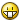How Do I Stop A Decimal number in Random

Recommended Posts

Dim \$word = ""
\$R = Random(1, 81520)
msgbox(4096, "", \$R )

How do I change this so the random number is not a decimal ? I just need it to be a whole number.

Share on other sites

thick

Dim \$word = ""
\$R = Int(Random(1, 81521))
msgbox(4096, "", \$R )

Int is a little better than Round since it is faster:

\$t = TimerInit()
For \$c = 1 to 100000
Int(Random(0,10))
Next
MsgBox(0,'',TimerDiff(\$t))

\$t = TimerInit()
For \$c = 1 to 100000
Round(Random(0,9))
Next
MsgBox(0,'',TimerDiff(\$t))
Edited by ezzetabi
Share on other sites

As usual, many thanks guys.

Share on other sites

In the beta version I've also added a flag to force INT values. It bugged me.Share on other sites

Well with your help I finished my sad password generator script. I know there are loads of free ones out there etc but it makes me feel better that the one thats gonna be used on the network has something of 'me' in it. :">

; AutoIt Version:  3.00

; Language:        English

; Platform:        WinXP

; Author:          Matt

; Script Function:  Password Generator from words file

\$__msgbox = MsgBox(4, 'Password Generator By Matt ', 'This Will Generate A Password For You, Continue?')

if (\$__msgbox = 7) Then

Exit

EndIf

Dim \$word = ""

\$R = Round(Random(1, 81520))

\$word = FileOpen("C:\words.txt", 0)

If \$word = -1 Then

MsgBox(0, "Error", "Unable to open file.")

Exit

EndIf

Sleep(3000)

FileClose(\$word)

SplashOff()

Create an account

Register a new account